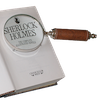#### You may also like### Double Digit### Number Detective

Follow the clues to find the mystery number.### Six Is the Sum

What do the digits in the number fifteen add up to? How many other numbers have digits with the same total but no zeros?

# The Number Jumbler

### Why do this problem?

The interactivity in this problem performs a trick in which the machine appears to read your mind and pick out the symbol you were thinking of. The mystery of the trick and the amazement when the machine gets it right time after time provide hooks to engage students' curiosity - there is an overwhelming need to explain the mystery and figure out what is going on!

Once students dig a little deeper and apply some mathematical thinking to the situation, the truth emerges - the mathematical steps of the trick force certain properties on the numbers chosen, so all the computer has to do is work within those parameters. Rather than spoiling the magic though, this shows students the power that mathematics has to explain the world, and gives them the tools they need to explore and create similar tricks of their own!

### Possible approach

Show the interactivity to the class, and read out the instructions to make sure everyone understands the process:

"Choose any two-digit number, add together both the digits and then subtract the total from your original number."
Once everyone has worked out their answer, invite them to find their symbol in the table on the right of the interactivity, and then click on the Number Jumbler machine. After a few tumbles, one picture will emerge from the machine. You could ask for a show of hands to see how many people were thinking of the same picture - it should be everyone!

Ask for suggestions as to how they think the 'trick' might work. Some might think that everyone picked the same number. Others might think it was just lucky. Others might have started to twig that there's some interesting mathematics going on...

Next, try out a few more examples. Students could pick two or three starting numbers and repeat the process. Then collect their answers together on the board. Once there is a conjecture that the answer is always a multiple of 9, return to the Number Jumbler to check whether multiples of 9 share an image.

To complete the understanding of the trick, students could take time to explore why the answer is always a multiple of 9. Here are some possible explanations they might come up with:

"Say you choose a number in the sixties. When you subtract the units, it goes down to 60 and then you take off another 6 for the tens digit which takes you to 54. If you were in the seventies you'd go down to 70 and then 63. I can check them all and it always gives a multiple of 9."

"I chose 34 which is 3 tens and 4 ones. When I added together the two digits and took them away, that's the same as taking away each digit separately. Taking away 4 leaves me with 30 which is 3 tens. Taking away 3 from 3 tens leaves me with 3 nines. It doesn't matter what the digits are, you'll always get a multiple of nine."

"I can use algebra to represent my two digit number. If it has $a$ in the tens column and $b$ in the units column, the number can be written $10a+b$. I then subtract $(a+b)$ from my total. $10a+b-(a+b)=9a$ so the answer is always a multiple of 9.

### Key questions

Which numbers in the grid have the same picture?
What do you notice about all the answers you get when you try a variety of starting points?
Why is "add together the digits and subtract the total from your original number" the same as "take away one digit and then take away the other"?

### Possible support

A good grasp of place value is really useful for understanding the trick in this problem. Two-digit Targets and Nice or Nasty could be useful for consolidating place value before working on the problem.

### Possible extension

Always a Multiple? offers a selection of suitable follow-up number tricks that can be explained using similar mathematical ideas.